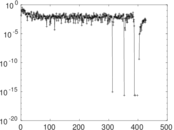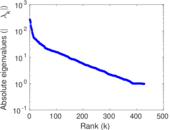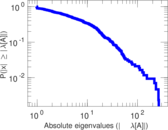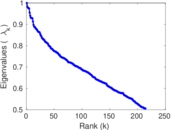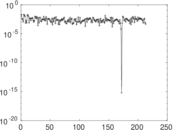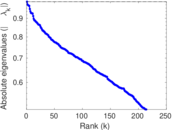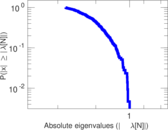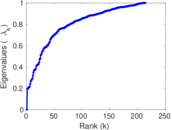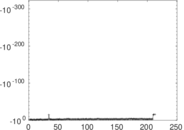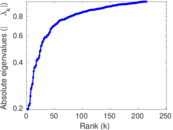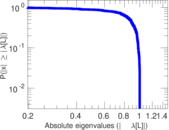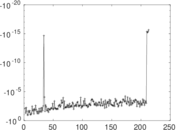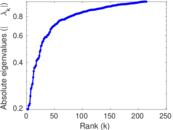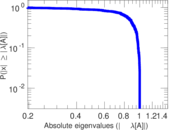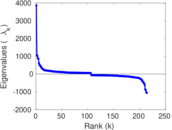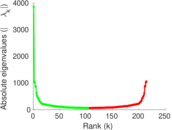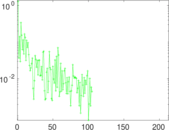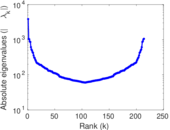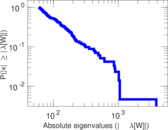# Wikipedia edits (tcy)

This is the bipartite edit network of the Tulu Wikipedia. It contains users and pages from the Tulu Wikipedia, connected by edit events. Each edge represents an edit. The dataset includes the timestamp of each edit.

 Code `tcy` Internal name `edit-tcywiki` Name Wikipedia edits (tcy) Data source http://dumps.wikimedia.org/ AvailabilityDataset is available for download Consistency checkDataset passed all tests Category Authorship network Dataset timestamp 2017-10-20 Node meaning User, article Edge meaning Edit Network formatBipartite, undirected Edge typeUnweighted, multiple edges Temporal dataEdges are annotated with timestamps

## Statistics

 Size n = 3,884 Left size n1 = 1,635 Right size n2 = 2,249 Volume m = 30,401 Unique edge count m̿ = 11,592 Wedge count s = 2,707,663 Claw count z = 807,134,041 Cross count x = 202,238,158,366 Square count q = 2,053,754 4-Tour count T4 = 27,293,148 Maximum degree dmax = 4,149 Maximum left degree d1max = 4,149 Maximum right degree d2max = 750 Average degree d = 15.654 5 Average left degree d1 = 18.593 9 Average right degree d2 = 13.517 6 Fill p = 0.003 152 47 Average edge multiplicity m̃ = 2.622 58 Size of LCC N = 3,823 Diameter δ = 8 50-Percentile effective diameter δ0.5 = 3.144 91 90-Percentile effective diameter δ0.9 = 4.613 68 Median distance δM = 4 Mean distance δm = 3.599 17 Gini coefficient G = 0.713 603 Balanced inequality ratio P = 0.233 364 Left balanced inequality ratio P1 = 0.125 588 Right balanced inequality ratio P2 = 0.270 616 Relative edge distribution entropy Her = 0.811 452 Power law exponent γ = 2.068 49 Tail power law exponent γt = 2.021 00 Tail power law exponent with p γ3 = 2.021 00 p-value p = 0.798 000 Left tail power law exponent with p γ3,1 = 2.001 00 Left p-value p1 = 0.495 000 Right tail power law exponent with p γ3,2 = 2.411 00 Right p-value p2 = 0.272 000 Degree assortativity ρ = −0.405 850 Degree assortativity p-value pρ = 0.000 00 Spectral norm α = 264.661 Algebraic connectivity a = 0.196 480 Spectral separation |λ1[A] / λ2[A]| = 1.015 29 Controllability C = 2,879 Relative controllability Cr = 0.742 968

## Plots

### Fruchterman–Reingold graph drawing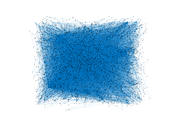### Degree distribution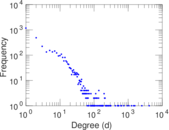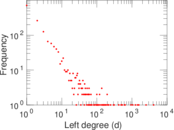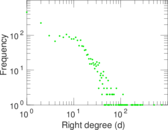### Cumulative degree distribution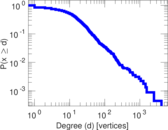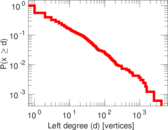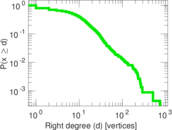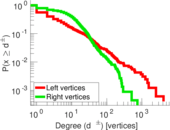### Lorenz curve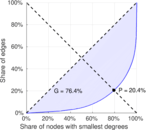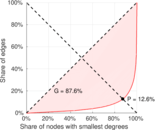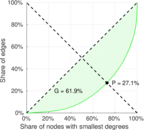### Spectral distribution of the adjacency matrix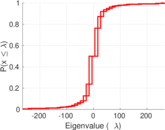### Spectral distribution of the normalized adjacency matrix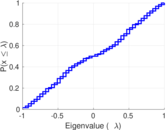### Spectral distribution of the Laplacian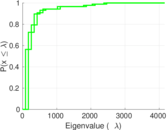### Spectral graph drawing based on the adjacency matrix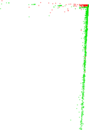### Spectral graph drawing based on the Laplacian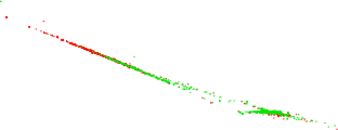### Spectral graph drawing based on the normalized adjacency matrix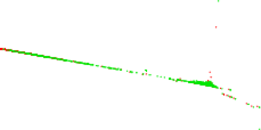### Degree assortativity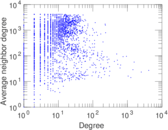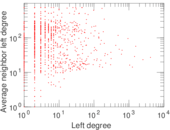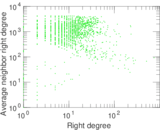### Zipf plot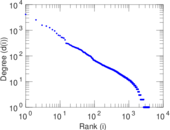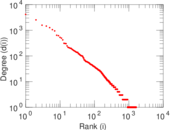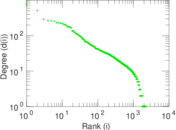### Hop distribution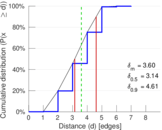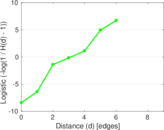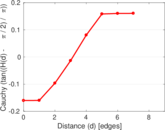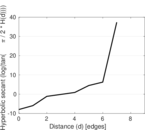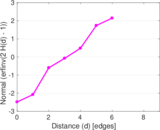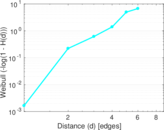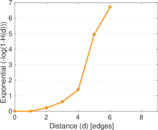### Double Laplacian graph drawing### Delaunay graph drawing### Edge weight/multiplicity distribution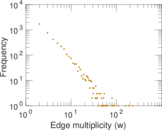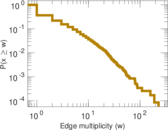### Temporal distribution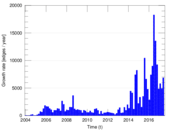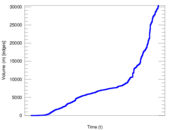### Temporal hop distribution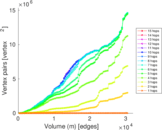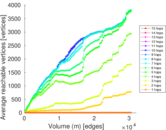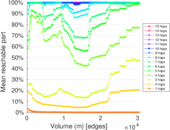### Diameter/density evolution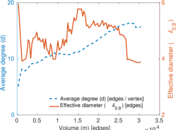### Matrix decompositions plots